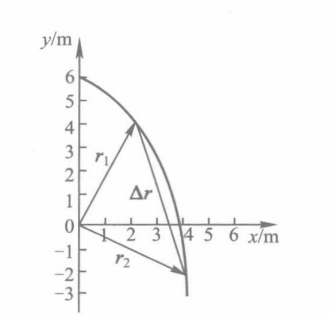$$x=2 t, y=6-2 t^2$$

$$y=6-\frac{x^2}{2}$$(2) 质点在 $t_1=1 \mathrm{~s}$ 和 $t_2=2 \mathrm{~s}$ 时的位矢分别为
\begin{aligned} & \boldsymbol{r}_1=(2 \boldsymbol{i}+4 \boldsymbol{j}) \mathrm{m} \\ & \boldsymbol{r}_2=(4 \boldsymbol{i}-2 \boldsymbol{j}) \mathrm{m} \end{aligned}

$$\Delta \boldsymbol{r}=\boldsymbol{r}_2-\boldsymbol{r}_1=(4 i-2 j) \mathrm{m}-(2 i+4 j) \mathrm{m}=(2 \boldsymbol{i}-6 j) \mathrm{m}$$

$$\begin{gathered} |\Delta \boldsymbol{r}|=\sqrt{(\Delta x)^2+(\Delta y)^2}=\sqrt{2^2+6^2} \mathrm{~m}=6.32 \mathrm{~m} \\ \theta=\arctan \frac{\Delta y}{\Delta x}=\arctan \frac{-6}{2}=-71.5^{\circ} \end{gathered}$$

\begin{aligned} \Delta|\boldsymbol{r}| & =\Delta r=r_2-r_1=\sqrt{x_2^2+y_2^2}-\sqrt{x_1^2+y_1^2} \\ & =\left[\sqrt{4^2+(-2)^2}-\sqrt{2^2+4^2}\right] \mathrm{m}=0 \end{aligned}

$$\bar{v}=\frac{|\Delta r|}{\Delta t}=\frac{6.32}{2-1} \mathrm{~m} / \mathrm{s}=6.32 \mathrm{~m} / \mathrm{s}$$

(3) 由运动学方程可得速度和加速度表示式
$$\begin{gathered} \boldsymbol{v}=\frac{\mathrm{d} \boldsymbol{r}}{\mathrm{d} t}=2 \boldsymbol{i}-4 t \boldsymbol{j} \\ \boldsymbol{a}=\frac{\mathrm{d} v}{\mathrm{~d} t}=-4 \boldsymbol{m} / \mathrm{s}^2 \\ t_1=1 \mathrm{~s} \text { 时 }: \boldsymbol{v}_1=(2 \boldsymbol{i}-4 \boldsymbol{j}) \mathrm{m} / \mathrm{s}, \quad a_1=-4 j \mathrm{~m} / \mathrm{s}^2 . \\ t_2=2 \mathrm{~s} \text { 时 }: \boldsymbol{v}_2=(2 \boldsymbol{i}-8 \boldsymbol{j}) \mathrm{m} / \mathrm{s}, \quad a_2=-4 j \mathrm{~m} / \mathrm{s}^2 . \end{gathered}$$

\begin{aligned} & v_1=\sqrt{2^2+(-4)^2} \mathrm{~m} / \mathrm{s}=4.47 \mathrm{~m} / \mathrm{s}, \quad \theta_1=\arctan \left(-\frac{4}{2}\right)=-63.5^{\circ} \\ & v_2=\sqrt{2^2+(-8)^2} \mathrm{~m} / \mathrm{s}=8.25 \mathrm{~m} / \mathrm{s}, \quad \theta_2=\arctan \left(\frac{-8}{2}\right)=-76.0^{\circ} \end{aligned}
$a_1=a_2=4 \mathrm{~m} / \mathrm{s}^2$, 沿 $O y$ 轴负方向.

(4) 质点离原点的距离就是位矢的量值, 即
$$r=|\boldsymbol{r}|=\sqrt{x^2+y^2}=\sqrt{(2 t)^2+\left(6-2 t^2\right)^2}$$

$$\frac{\mathrm{d} r}{\mathrm{~d} t}=\frac{4 t\left(2 t^2-5\right)}{\sqrt{(2 t)^2+\left(6-2 t^2\right)}}=0$$

$$4 t\left(2 t^2-5\right)=0$$
$t=0$ 或 $t=\sqrt{\frac{5}{2}} \mathrm{~s}=1.58 \mathrm{~s}, t=-1.58 \mathrm{~s}$ (舍去)

①点击 收藏 此题， 扫码注册关注公众号接收信息推送（一月四份试卷,中1+高2+研1）
② 程序开发、服务器资源都需要大量的钱，如果你感觉本站好或者受到到帮助，欢迎赞助本站,赞助方式：微信/支付宝转账到 18155261033

 ①此题难易度如何 ②此题推荐度如何 确定